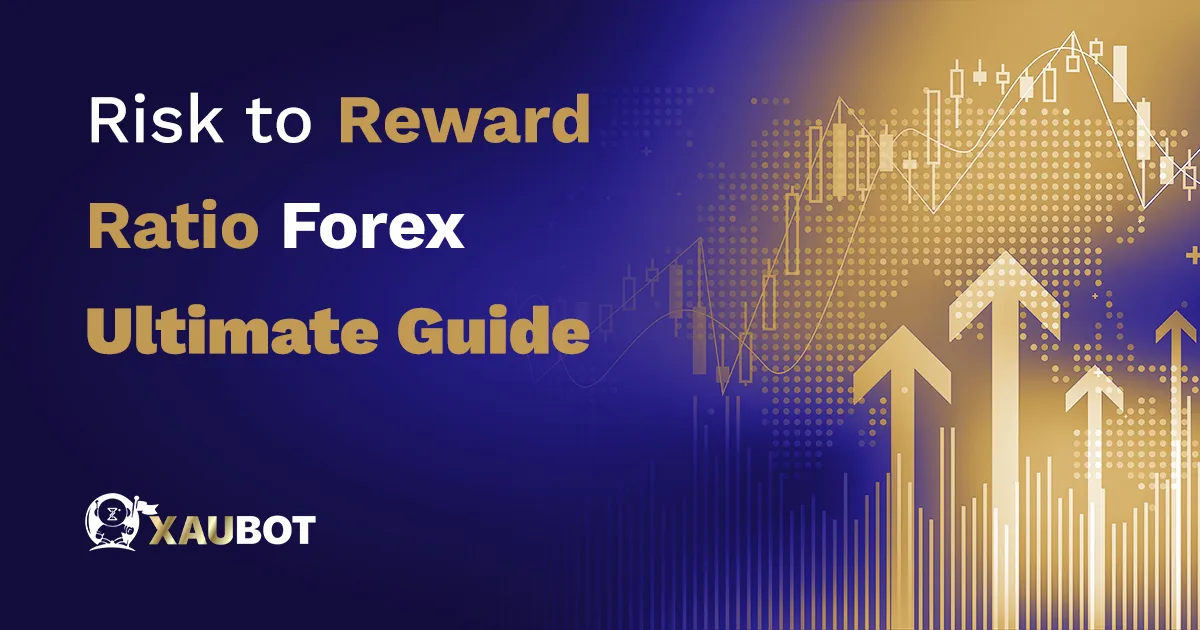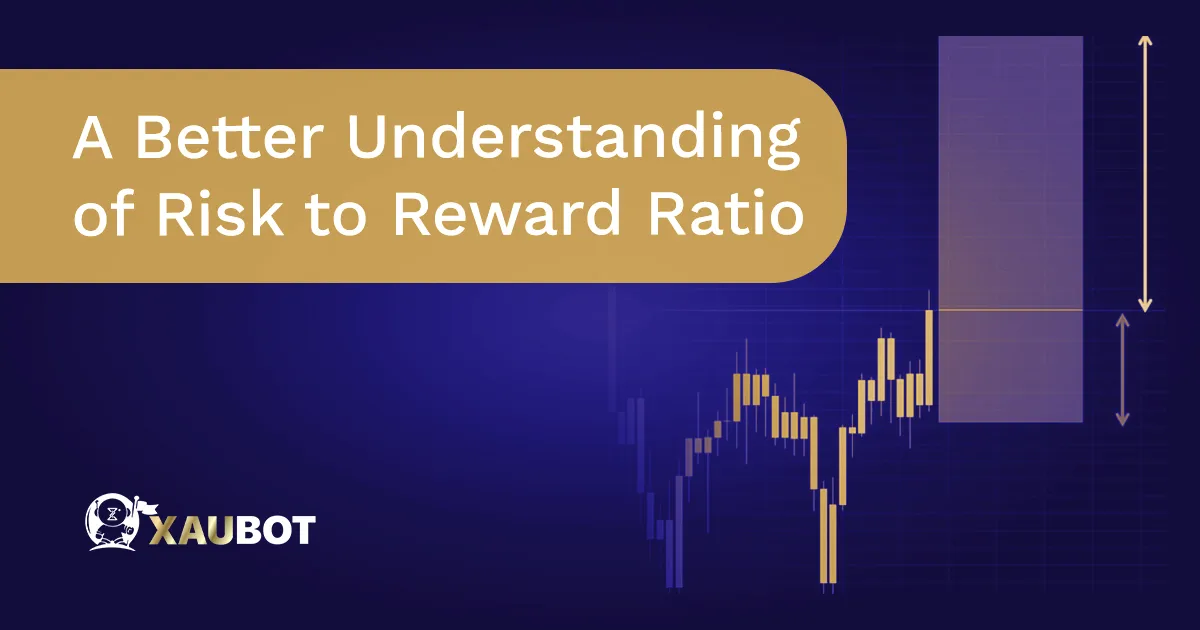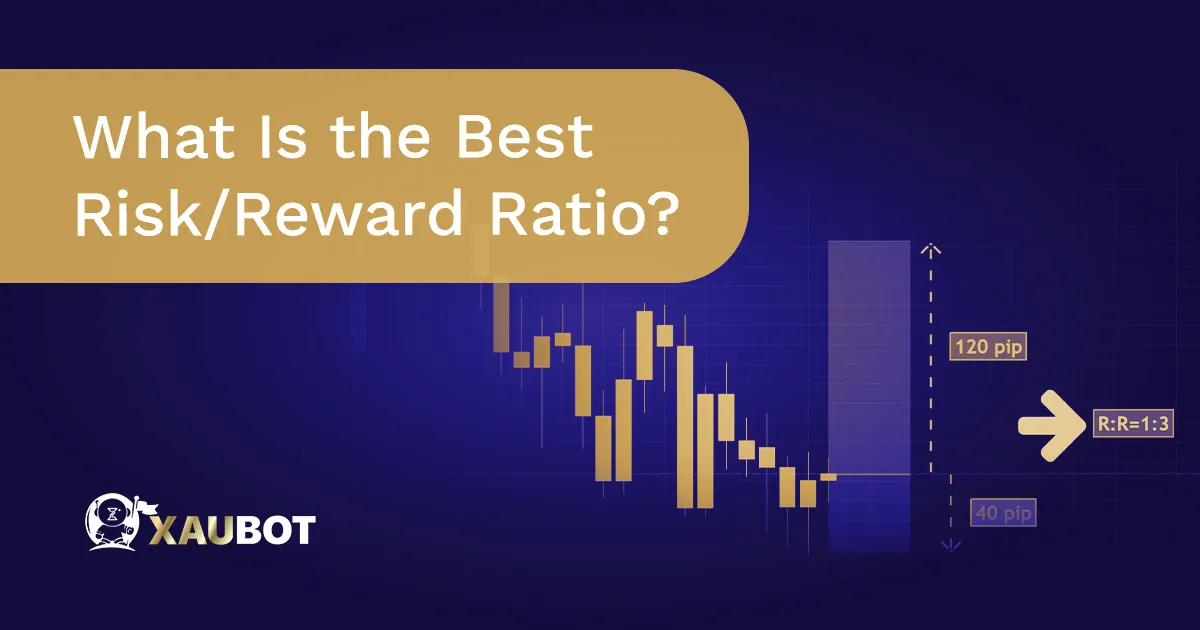Risk to Reward Ratio Forex Ultimate Guide - XAUBOT

# Risk to Reward Ratio Forex Ultimate GuideContents

Risk management is a key component of forex trading. It is the notion that leads to sustainable profit margins through trading in this volatile and heavily active market.

But risk management as a whole is an umbrella term that entails a large number of tools and techniques. These concepts related to managing risks ensure that you as a trader can keep on earning and avoid any unnecessary loss. Now, keep it in mind that loss is at time unavoidable in the market. It is part of trading. But unnecessary loss should be avoided altogether.

The notion of risk to reward ratio is precisely developed to provide traders with a concrete figure and ratio in order to measure the effectiveness of their risk management.

In this article, we want to provide you with an ultimate guide on risk/reward ratio in the forex market.

## A Better Understanding of Risk to Reward RatioA Better Understanding of Risk to Reward Ratio

On the face of it, one can understand that risk to reward ratio is the proportion of the profits you gain to the losses you incur.

But a better understanding of this ratio would be how much profit or return you obtain for the amount of assets or funds that you put in the market.

The account currency denomination and also the currency pair denomination are important in calculating and considering this ratio. But supposing that the main denomination is the US dollar, we can provide you with an example.

If you have a risk to reward ratio of 1 to 4 or (1:4), this means that you risk 1 dollar in order to make 4 dollars. Of course this is only a simple illustration of this ratio. The precise numbers depend upon the lot size and even stop loss levels, among others.

## Is There an Ideal Risk to Reward Ratio in Forex?

There are so many experts and sources that claim to have found the magical ratio for risk to reward. The most commonly referred to ratio is 1:2. Which basically means for every dollar, you can potentially win two dollars.

But the point is, in order to obtain the most useful data from risk to reward ratio, we also need to consider your win rate in the market.

For example, if you have a win rate of 30% in your trades, even with a risk to reward ratio of 1:2, you will be losing money. How? Let’s illustrat:

Win rate: 30% | Risk to reward ratio: 1:2

In this situation, out of every 10 trades, you will lose and gain the following:

How much you lose: \$1 × 7 (losing trades) = -\$7

How much you gain: \$2 × 3 (winning trades) = \$6

So even with a risk to reward ratio of 1:2 you are losing more than you are gaining with a win rate of 30%. Therefore, it is quite clear that this ratio on its own is not the final word on determining the outcome of your trades.

Though, coupled with other factors and indices, including win rate, we can get a much clearer picture.

## How to Avoid Losing Money with Risk to Reward Ratio?

We saw that the risk to reward ratio on its own is almost misleading. Don’t get it wrong, this ratio holds extremely valuable information and data regarding your trading approach and results.

But let’s see, using some precise examples, how you can use your risk to reward ratio in order to actually avoid losses and obtain profits sustainably.

Given your risk to reward ratio, you need to adjust your win rate. For instance, with a ratio of 1:5 you need to have at least a win rate of 20% in order to stay afloat and above water. There with a ratio of 1:5 and 20%-win rate:

How much you lose: \$1 × 8 (losing trades) = -\$8

How much you gain: \$5 × 2 (winning trades) = \$10

So you can see with this minimum win rate you can keep a net positive outcome for your trades. You can easily calculate this minimum win rate yourself for any risk to reward ratio. But in general, for the following ratios we can have the mentioned minimum win rate:

Risk/Reward 1:2 = 40% minimum win rate

Risk/Reward 1:3 = 30% minimum win rate

Risk/Reward 1:4 = between 20% to 30% minimum win rate

Although these are rough numbers. And you also need to consider broker fees and commissions that are also part of every trade.

## What Is the Best Risk/Reward Ratio?What Is the Best Risk/Reward Ratio?

After all that has been said, should you look for a risk to reward ratio that works best in forex trading? Well, the answer to this intriguing question is largely dependent upon your available funds for trading and your trading style and strategy.

So, let’s set out some actual examples and go into the specifics. But before we do that, we need to recap the notions of risk and reward and their proportionality with regard to one another.

We can define risk as the maximum amount that can be lost in any given trade. And reward is the maximum amount that can be gained from any given trade. Then in this sense, the risk to reward ratio is the proportion of these two concepts.

Naturally, what can be understood from these notions, is that whether you are a beginner or an experienced trader, or whether you are a day trader or a position trader, all of these are contributing factors.

So, for example small ratios such as 1:3 or 1:4 would be much more ideal for beginner traders as they are easier to obtain and uphold in the long run without sustaining losses.

But for a much more experienced and skilled trader, a high ratio such as 1:10 would be ideal, where for every dollar, you can potentially earn 10 dollars.

##### Conclusion

The risk to reward ratio is one of the most well-known figures among all forex traders. It will tell you how much you can gain in the grand scheme of your trades. There are so many wrong notions and misunderstandings with regard to the risk reward ratio out there.

Whether you have the right ratio or not will ultimately depend upon your trading style and approach. This could mean that even a ratio of 1:3 could potentially translate to winning \$9,000 against a loss of \$3,000 which means a net profit of \$6,000 in total.

Although, you should always consider the risk to reward ratio as a dynamic notion that is impacted by other factors. Together with other crucially important concepts, such as the stop loss level, all of these methods can be used to maximally manage risks associated with trading in the forex market.

Share Posts
You may also like